Home » A Comprehensive Guide to 21 Popular Deep Learning Interview Questions and Answers

# A Comprehensive Guide to 21 Popular Deep Learning Interview Questions and Answers

## Overview

• Looking to crack your next deep learning interview? You’ve come to the right place!
• We have put together a list of popular deep learning interview questions in this article
• Each question comes with a comprehensive answer as well to guide you

## Introduction

Are you planning to sit for deep learning interviews? Have you perhaps already taken the first step, applied, and sat through the ordeal of several rounds of interviews for a deep learning role and not made the cut?

Cracking an interview, especially for a complex role like a deep learning specialist, is a daunting task for most people. Deep learning is a vast field with an ever-changing nature as new developments are rolled out on a regular basis. How can you keep up with the pace? What should you focus on?

These are questions every deep learning enthusiast, fresher and even expert has asked themselves at some point.That was a key reason behind penning down this article, a comprehensive list of the popular deep learning interview questions and answers. But let me expand on that a bit more.

Note: Make sure you check out the popular Fundamentals of Deep Learning course if you harbor any deep learning career ambitions!

## Why Have We Created this List of Deep Learning Questions?

There are plenty of resources on the internet about Machine Learning and Data Science Interviews. The rapid rise of Machine Learning and the solutions it provides to complex tasks has been remarkable and the industry has responded to this rise really well. Almost every big company has a Data Science Team, and almost every other startup leverages Machine Learning for its product.

However, we are now seeing a tectonic shift in the industry. As the need for more complex solutions grows around the world, organizations are turning to deep learning frameworks. Advances in computer vision and natural language processing (NLP) have created a need to adopt deep learning or stay behind the curve.

The demand for deep learning folks is growing every month! This is a great time to polish your skills and start climbing the deep learning hill.

My aim here is to make this article simple and to-the-point while explaining the core ideas behind the questions. I have also listed resources where you can learn more about the deep learning topics in the questions.

Also, this does not mean that your interview will not contain a single question on Machine Learning. There are some concepts that are common in both fields and are extremely crucial for you to know. These include topics like:

• Evaluation Metrics
• Bias vs. Variance (or Underfitting vs .Overfitting)
• Cross-validation, etc.

So, here is a definitive interview guide that covers all the topics in details, in the form of MCQs and long-form resources: The Most Comprehensive Data Science & Machine Learning Interview Guide You’ll Ever Need. If you’re looking for a structured and granular guide including tips, tricks and case studies on how to crack interviews, I highly recommend taking the Ace Data Science Interviews course.

In this comprehensive guide, I have organized the deep learning questions into three levels:

• Beginner
• Intermediate

There’s something here for everyone! So get your pen and paper ready, strap in, and prepare to learn.

## Beginner-Level Deep Learning Interview Questions

These questions are typically asked to make the candidate familiar with the interviewer and the interview setting. While the questions themselves may not be very difficult to answer, this level is your best chance to convince the interviewer that your fundamental concepts around deep learning are clear.

### 1. What is the difference between a Perceptron and Logistic Regression?

A Multi-Layer Perceptron (MLP) is one of the most basic neural networks that we use for classification. For a binary classification problem, we know that the output can be either 0 or 1. This is just like our simple logistic regression, where we use a logit function to generate a probability between 0 and 1.

So, what’s the difference between the two?

Simply put, it is just the difference in the threshold function! When we restrict the logistic regression model to give us either exactly 1 or exactly 0, we get a Perceptron model: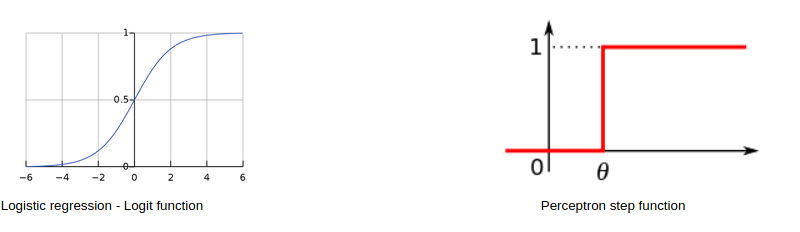### 2. Can we have the same bias for all neurons of a hidden layer?

Essentially, you can have a different bias value at each layer or at each neuron as well. However, it is best if we have a bias matrix for all the neurons in the hidden layers as well.

A point to note is that both these strategies would give you very different results.

### 3. What if we do not use any activation function(s) in a neural network?

The main aim of this question is to understand why we need activation functions in a neural network. You can start off by giving a simple explanation of how neural networks are built:

Step 1: Calculate the sum of all the inputs (X) according to their weights and include the bias term:

Z = (weights * X) + bias

Step 2: Apply an activation function to calculate the expected output:

Y = Activation(Z)

Steps 1 and 2 are performed at each layer. If you recollect, this is nothing but forward propagation! Now, what if there is no activation function?

Our equation for Y essentially becomes:

Y = Z = (weights * X) + bias

Wait – isn’t this just a simple linear equation? Yes – and that is why we need activation functions. A linear equation will not be able to capture the complex patterns in the data – this is even more evident in the case of deep learning problems.

In order to capture non-linear relationships, we use activation functions, and that is why a neural network without an activation function is just a linear regression model.

### 4. In a neural network, what if all the weights are initialized with the same value?

In simplest terms, if all the neurons have the same value of weights, each hidden unit will get exactly the same signal. While this might work during forward propagation, the derivative of the cost function during backward propagation would be the same every time.

In short, there is no learning happening by the network! What do you call the phenomenon of the model being unable to learn any patterns from the data? Yes, underfitting.

Therefore, if all weights have the same initial value, this would lead to underfitting.

Note: This question might further lead to questions on exploding and vanishing gradients, which I have covered below.

### 5. List the supervised and unsupervised tasks in Deep Learning.

Now, this can be one tricky question. There might be a misconception that deep learning can only solve unsupervised learning problems. This is not the case. Some example of Supervised Learning and Deep learning include:

• Image classification
• Text classification
• Sequence tagging

On the other hand, there are some unsupervised deep learning techniques as well:

Here is a great article on applications of Deep Learning for unsupervised tasks:

### 6. What is the role of weights and bias in a neural network?

This is a question best explained with a real-life example. Consider that you want to go out today to play a cricket match with your friends. Now, a number of factors can affect your decision-making, like:

• How many of your friends can make it to the game?
• How much equipment can all of you bring?
• What is the temperature outside?

And so on. These factors can change your decision greatly or not too much. For example, if it is raining outside, then you cannot go out to play at all. Or if you have only one bat, you can share it while playing as well. The magnitude by which these factors can affect the game is called the weight of that factor.

Factors like the weather or temperature might have a higher weight, and other factors like equipment would have a lower weight.

However, does this mean that we can play a cricket match with only one bat? No – we would need 1 ball and 6 wickets as well. This is where bias comes into the picture. Bias lets you assign some threshold which helps you activate a decision-point (or a neuron) only when that threshold is crossed.

### 7. How does forward propagation and backpropagation work in deep learning?

Now, this can be answered in two ways. If you are on a phone interview, you cannot perform all the calculus in writing and show the interviewer. In such cases, it best to explain it as such:

• Forward propagation: The inputs are provided with weights to the hidden layer. At each hidden layer, we calculate the output of the activation at each node and this further propagates to the next layer till the final output layer is reached. Since we start from the inputs to the final output layer, we move forward and it is called forward propagation
• Backpropagation: We minimize the cost function by its understanding of how it changes with changing the weights and biases in a neural network. This change is obtained by calculating the gradient at each hidden layer (and using the chain rule). Since we start from the final cost function and go back each hidden layer, we move backward and thus it is called backward propagation

For an in-person interview, it is best to take up the marker, create a simple neural network with 2 inputs, a hidden layer, and an output layer, and explain it.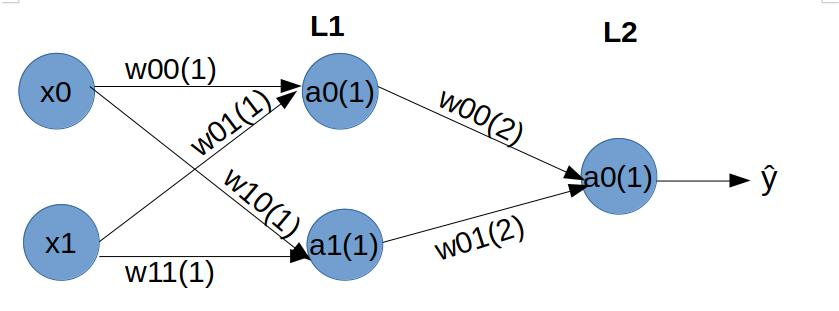Forward propagation: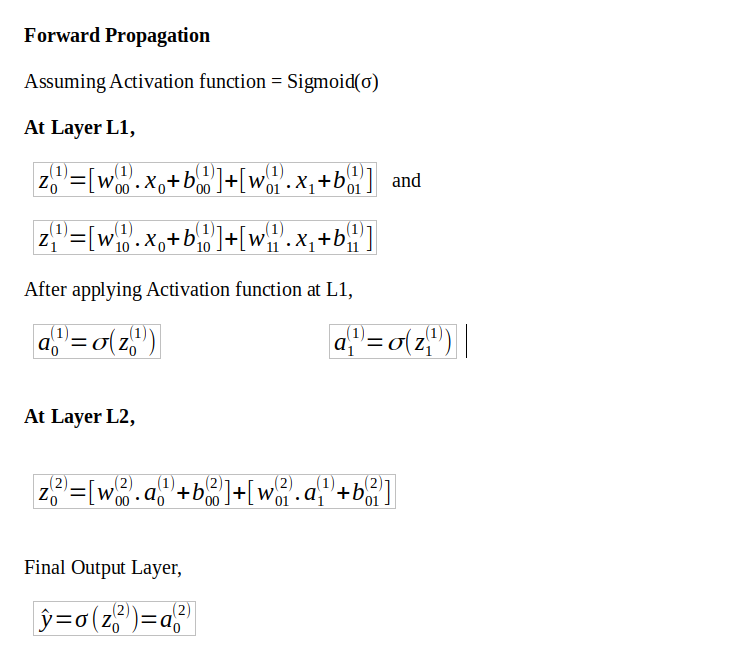Backpropagation:

At layer L2, for all weights: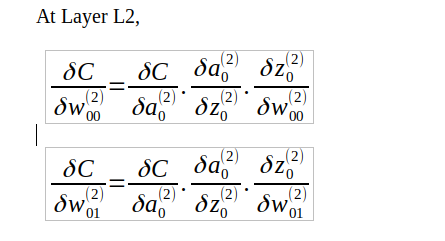At layer L1, for all weights: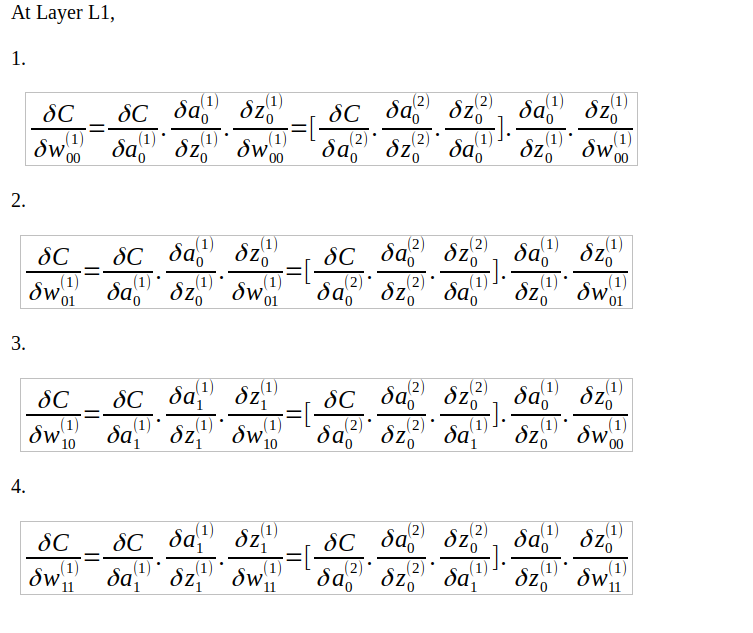You need not explain with respect to the bias term as well, though you might need to expand the above equations substituting the actual derivatives.

### 8. What are the common data structures used in Deep Learning?

Deep Learning goes right from the simplest data structures like lists to complicated ones like computation graphs.

Here are the most common ones:

• List: An ordered sequence of elements (You can also mention NumPy ndarrays here)
• Matrix: An ordered sequence of elements with rows and columns
• Dataframe: A dataframe is just like a matrix, but it holds actual data with the column names and rows denoting each datapoint in your dataset. If marks of 100 students, their grades, and their details are stored in a dataframe, their details are stored as columns. Each row will represent the data of each of the 100 students
• Tensors: You will work with them on a daily basis if you have ventured into deep learning. Used both in PyTorch and TensorFlow, tensors are like the basic programming unit of deep learning. Just like multidimensional arrays, we can perform numerous mathematical operations on them. Read more about tensors here
• Computation Graphs: Since deep learning involves multiple layers and often hundreds, if not thousands of parameters, it is important to understand the flow of computation. A computation graph is just that. A computation graph gives us the sequence of operations performed with each node denoting an operation or a component in the neural network

## Intermediate-Level Deep Learning Interviews Questions

Once the basics are out of the way, the interview would lead to slightly advanced deep learning concepts. These questions are much easier to answer if you have considerable practice with not only the mathematical concepts but also with coding them.

Additionally, these questions can also become more project-specific. As a general rule of thumb, it is best to include examples of how you have used the concept asked in the question in your own projects. This has two advantages:

1. It will let the interviewer know that you have practical experience as well
2. Since you are talking about projects that you have implemented, it is much easier and comfortable to talk about your own work

Here, I have given an overview of the key concepts in the questions – you can always customize your answers to add more about your experiences with some of these deep learning algorithms and techniques.

### 9. Why should we use Batch Normalization?

Once the interviewer has asked you about the fundamentals of deep learning architectures, they would move on to the key topic of improving your deep learning model’s performance.

Batch Normalization is one of the techniques used for reducing the training time of our deep learning algorithm. Just like normalizing our input helps improve our logistic regression model, we can normalize the activations of the hidden layers in our deep learning model as well: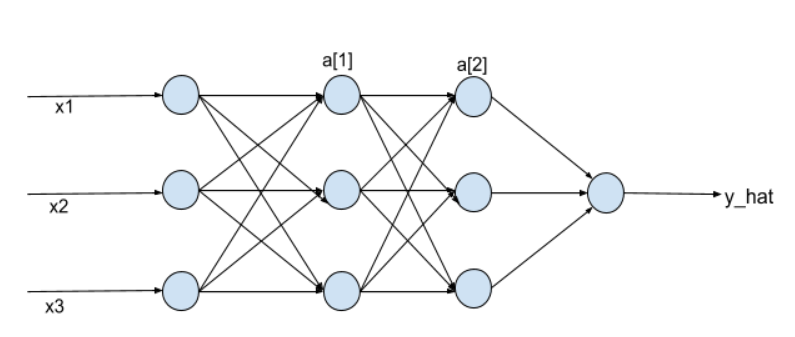We basically normalize a and a here. This means we normalize the inputs to the layer, and then apply the activation functions to the normalized inputs.

Here is an article that explains Batch Normalization and other techniques for improving Neural Networks: Neural Networks – Hyperparameter Tuning, Regularization & Optimization.

### 10. List the activation functions you have used so far in your projects and how you would choose one.

The most common activation functions are:

• Sigmoid
• Tanh
• ReLU
• Softmax

While it is not important to know all the activation functions, you can always score points by knowing the range of these functions and how they are used. Here is a handy table for you to follow: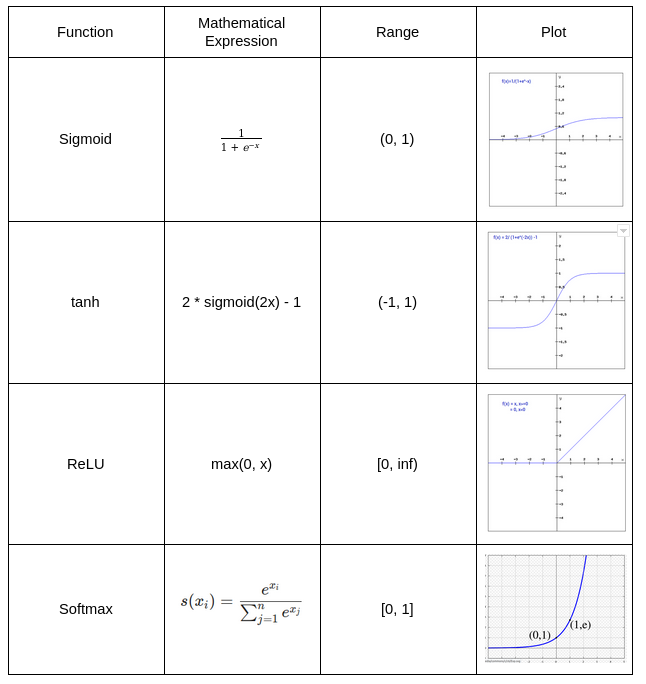Here is a great guide on how to use these and other activations functions: Fundamentals of Deep Learning – Activation Functions and When to Use Them?.

### 11. Why does a Convolutional Neural Network (CNN) work better with image data?

The key to this question lies in the Convolution operation. Unlike humans, the machine sees the image as a matrix of pixel values. Instead of interpreting a shape like a petal or an ear, it just identifies curves and edges.

Thus, instead of looking at the entire image, it helps to just read the image in parts. Doing this for a 300 x 300 pixel image would mean dividing the matrix into smaller 3 x 3 matrices and dealing with them one by one. This is convolution.

Mathematically, we just perform a small operation on the matrix to help us detect features in the image – like boundaries, colors, etc.

Z = X * f

Here, we are convolving (* operation – not multiplication) the input matrix X with another small matrix f, called the kernel/filter to create a new matrix Z. This matrix is then passed on to the other layers.

If you have a board/screen in front of you, you can always illustrate this with a simple example: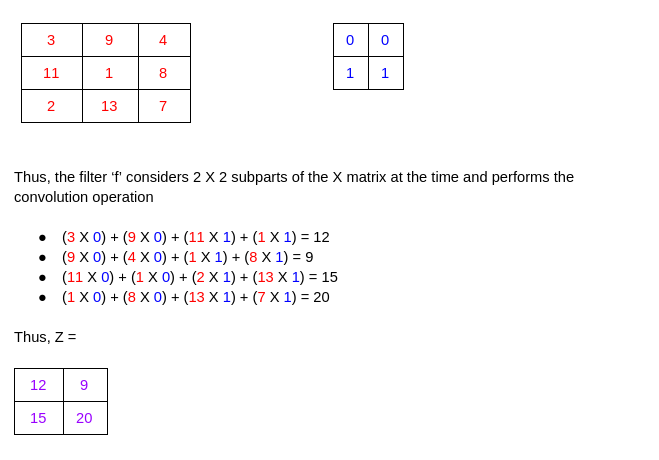Learning more about how CNNs work here.

### 12. Why do RNNs work better with text data?

The main component that differentiates Recurrent Neural Networks (RNN) from the other models is the addition of a loop at each node. This loop brings the recurrence mechanism in RNNs. In a basic Artificial Neural Network (ANN), each input is given the same weight and fed to the network at the same time. So, for a sentence like “I saw the movie and hated it”, it would be difficult to capture the information which associates “it” with the “movie”.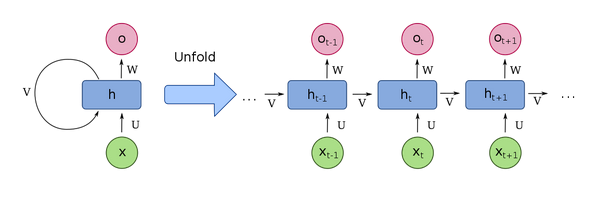The addition of a loop is to denote preserving the previous node’s information for the next node, and so on. This is why RNNs are much better for sequential data, and since text data also is sequential in nature, they are an improvement over ANNs.

### 13. In a CNN, if the input size 5 X 5 and the filter size is 7 X 7, then what would be the size of the output?

This is a pretty intuitive answer. As we saw above, we perform the convolution on ‘x’ one step at a time, to the right, and in the end, we got Z with dimensions 2 X 2, for X with dimensions 3 X 3.

Thus, to make the input size similar to the filter size, we make use of padding – adding 0s to the input matrix such that its new size becomes at least 7 X 7. Thus, the output size would be using the formula:

Dimension of image = (n, n) = 5 X 5

Dimension of filter = (f,f)  = 7 X 7

Padding = 1 (adding 1 pixel with value 0 all around the edges)

Dimension of output will be (n+2p-f+1) X (n+2p-f+1) = 1 X 1

### 14. What’s the difference between valid and same padding in a CNN?

This question has more chances of being a follow-up question to the previous one. Or if you have explained how you used CNNs in a computer vision task, the interviewer might ask this question along with the details of the padding parameters.

• Valid Padding: When we do not use any padding. The resultant matrix after convolution will have dimensions (n – f + 1) X (n – f + 1)
• Same padding: Adding padded elements all around the edges such that the output matrix will have the same dimensions as that of the input matrix

### 15. What do you mean by exploding and vanishing gradients?

The key here is to make the explanation as simple as possible. As we know, the gradient descent algorithm tries to minimize the error by taking small steps towards the minimum value. These steps are used to update the weights and biases in a neural network.

However, at times, the steps become too large and this results in larger updates to weights and bias terms – so much so as to cause an overflow (or a NaN) value in the weights. This leads to an unstable algorithm and is called an exploding gradient.

On the other hand, the steps are too small and this leads to minimal changes in the weights and bias terms – even negligible changes at times. We thus might end up training a deep learning model with almost the same weights and biases each time and never reach the minimum error function. This is called the vanishing gradient.

A point to note is that both these issues are specifically evident in Recurrent Neural Networks – so be prepared for follow-up questions on RNN!

### 16. What are the applications of transfer learning in Deep Learning?

I am sure you would have a doubt as to why a relatively simple question was included in the Intermediate Level. The reason is the sheer volume of subsequent questions it can generate!

The use of transfer learning has been one of the key milestones in deep learning. Training a large model on a huge dataset, and then using the final parameters on smaller simpler datasets has led to defining breakthroughs in the form of Pretrained Models. Be it Computer Vision or NLP, pretrained models have become the norm in research and in the industry.

Some popular examples include BERT, ResNet, GPT-2, VGG-16, etc and many more.

It is here that you can earn brownie points by pointing out specific examples/projects where you used these models and how you used them as well.

It is not possible to discuss all of them, so here are a few resources to get started:

## Advanced-Level Deep Learning Interview Questions

It is here that questions become really specific to your projects or to what you have discussed in the interview before.

Also, depending on the domain – with Computer Vision or Natural Language Processing, these questions can change. While it is not important to know the architecture of each model in detail, you would need to know the intuition behind them and why these models were needed in the first place.

Again, just like the intermediate level, it is important to always bring in examples that you have studied or implemented yourself into the discussion.

### 17. How backpropagation is different in RNN compared to ANN?

In Recurrent Neural Networks, we have an additional loop at each node:This loop essentially includes a time component into the network as well. This helps in capturing sequential information from the data, which could not be possible in a generic artificial neural network.

This is why the backpropagation in RNN is called Backpropagation through Time, as in backpropagation at each time step.

You can find a detailed explanation of RNNs here: Fundamentals of Deep Learning – Introduction to Recurrent Neural Networks.

### 18. How does LSTM solve the vanishing gradient challenge?

The LSTM model is considered a special case of RNNs. The problems of vanishing gradients and exploding gradients we saw earlier are a disadvantage while using the plain RNN model.

In LSTMs, we add a forget gate, which is basically a memory unit that retains information that is retained across timesteps and discards the other information that is not needed. This also necessitates the need for input and output gates to include the results of the forget gate as well.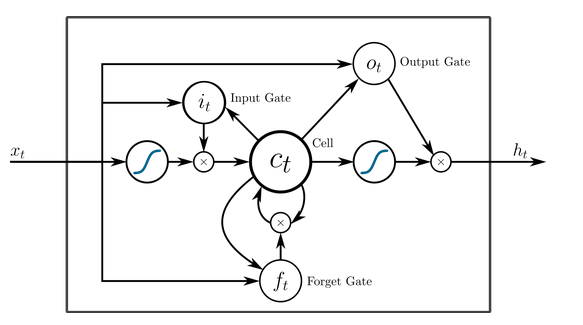### 19. Why is GRU faster as compared to LSTM?

As you can see, the LSTM model can become quite complex. In order to still retain the functionality of retaining information across time and yet not make a too complex model, we need GRUs.

Basically, in GRUs, instead of having an additional Forget gate, we combine the input and Forget gates into a single Update Gate: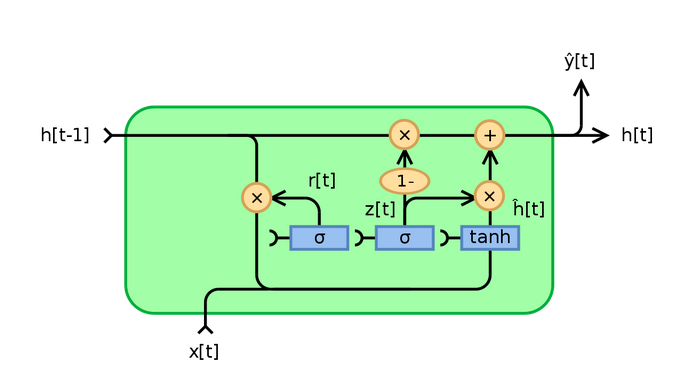It is this reduction in the number of gates that makes GRU less complex and faster than LSTM. You can learn about GRUs, LSTMs and other sequence models in detail here: Must-Read Tutorial to Learn Sequence Modeling & Attention Models.

### 20. How is the transformer architecture better than RNN?

Advancements in deep learning have made it possible to solve many tasks in Natural Language Processing. Networks/Sequence models like RNNs, LSTMs, etc. are specifically used for this purpose – so as to capture all possible information from a given sentence, or a paragraph. However, sequential processing comes with its caveats:

• It requires high processing power
• It is difficult to execute in parallel because of its sequential nature

This gave rise to the Transformer architecture. Transformers use what is called the attention mechanism. This basically means mapping dependencies between all the parts of a sentence.

Here is an excellent article explaining transformers: How do Transformers Work in NLP? A Guide to the Latest State-of-the-Art Models.

### 21. Describe a project you worked on and the tools/frameworks you used?

Now, this is one question that is sure to be asked even if none of the above ones is asked in your deep learning interview. I have included it in the advanced section since you might be grilled on each and every part of the code you have written.

Before the interview, make sure to:

• have your GitHub code updated with the latest code changes you have made
• be ready to give in-depth explanations on at least 2-3 projects where you used deep learning

When you are asked such a question, it is best to give a small 30-second pitch on what was the:

• problem statement
• data you used and the framework (like PyTorch or TensorFlow)
• any pretrained model you used or just the name of the basic model you built upon
• the value of the evaluation metric you achieved

After this, you can start going into detail about the model architecture, what preprocessing steps you had to take, and how that changed the data.

An important point to be noted is that the project need not be a very complicated or sophisticated one. A well-explained object detection project would earn you more points than a poorly-explained video classification project. Towards this end, I recommend having a README file in the above format for every project that you have implemented.

## End Notes

These are a list of a few key questions that you would come across in a deep learning interview. I have tried to cover more generic topics rather than go into the details of how deep learning is used in fields like NLP or computer vision.

I would always recommend to have a clear knowledge of the job description and prepare accordingly. If the role is more geared towards Computer Vision, I would recommend studying more on Convolutional Neural Networks and OpenCV, while Natural Language Processing roles would veer more towards RNNs, Transformers, etc.

I would love to hear your own interview experiences. Share them with me and the community in the comments section below.

You can also read this article on our Mobile APP•Victor says:
•AJAY VISHWAKARMA says: